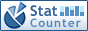Home PC Games Linux Windows Database Network Programming Server Mobile
 Home \ Programming \ Python closure and function objects - HAProxy performance under high concurrency (Server) - To use yum install VLC under CentOS (Linux) - Why I do not like the Go language style interface (ie Structural Typing) (Programming) - Linux install Maven and SVN client (Linux) - Ubuntu 13.04 configure MyEclipse 10.7 Environment (Linux) - Detailed usage history command (Linux) - MD5 and simple to use (Linux) - Linux delete duplicate files Artifact: dupeGuru (Linux) - High-performance JavaScript DOM programming (Programming) - Ubuntu 15.04 installed Nvidia Quadro series graphics driver (Linux) - Bash environment is automatically install and initialize oh-my-zsh & autojump zsh (Linux) - MySQL Basic tutorial: About varchar (N) (Database) - X security settings in Ubuntu (Linux) - Why is the ibdata1 file growing in MySQL? (Database) - Job achieve automation in Ubuntu 14.04 - Using Cron (Linux) - C language function pointer and a callback function (Programming) - Python substring format (Programming) - Ubuntu Apache virtual host configuration (Server) - Redis Design and Implementation study notes (Database) - C ++ class implementation date operator overloading (Programming) Python closure and function objects Add Date : 2018-11-21 1. Python What is closure The concept of function closures in python, this concept is what does it mean to see Wikipedia's explanation is as follows: In programming languages, closures (also lexical closures or function closures) are a technique for implementing lexically scoped name binding in languages ​​with first-class functions Operationally, a closure is a record storing a function [a] together with an environment:. [1 ] a mapping associating each free variable of the function (variables that are used locally, but defined in an enclosing scope) with the value or storage location to which the name was bound when the closure was created. [b] A closure-unlike a plain function-allows the function to access those captured variables through the closure's reference to them, even when the function is invoked outside their scope. The concept looks a lot, let's talk about the popular Suppose I have a request as a function of x ^ n def pow (x, n):     res = 1     for i in range (n):         res * = x     return res (example 1) In a piece of code, I always use the sum of the squares (for example, when seeking the rectangle diagonal), that my code is len2d = pow (20,2) + pow (30,2) I hope this time the second parameter always take two, not rewritable In another piece of code, I always use the cube and (for example, when seeking the cube diagonal), then I turned into code len3d = pow (20,3) + pow (30,3) + pow (40,3) I hope this time the second parameter is fixed at 3 In both cases above, the function closure comes into play: def pown (n):     def pow (x):         res = 1         for i in range (n): # function peripheral reference state             res * = x         return res     return pow pow2 = pown (2) len2d = pow2 (20) + pow2 (30)   pow3 = pown (3) len3d = pow3 (20) + pow3 (30) + pow3 (40) (Example 2) From Example 2 we see that, pown is a peripheral function, passing it a parameter n, and returns an internal function. pow is python closures function, it not only has its own implementation of logic, but also references to the parameter n. This is the closure function and a normal function of the biggest difference, in addition to the closure function performs the function body, and "closure" peripheral status. Each closure function instance can "closure" respective states. 2. Closures and function objects If you want to closures and c ++ to be a contrast, it should be similar to the c ++ function object. The code function object with the python to achieve the following: class Pow (object):     def __init __ (self, n):         self.n = n     def __call __ (self, x):         res = 1         for i in range (self.n): # reference object members             res * = x         return res pow2 = Pow (2) len2d = pow2 (20) + pow2 (30) pow3 = Pow (3) len3d = pow3 (20) + pow3 (30) + pow3 (40) (Example 3) Example Class 3 defines a special method __call__, so it can be done directly target function calls, called function objects. Since it is an object, so the initialization time can pass parameters to save, before this point is similar to the concept mentioned closures. In this analogy, the closures can be seen as a simplified approximation of a function of an object More:
 CopyRight 2002-2020 newfreesoft.com, All Rights Reserved.